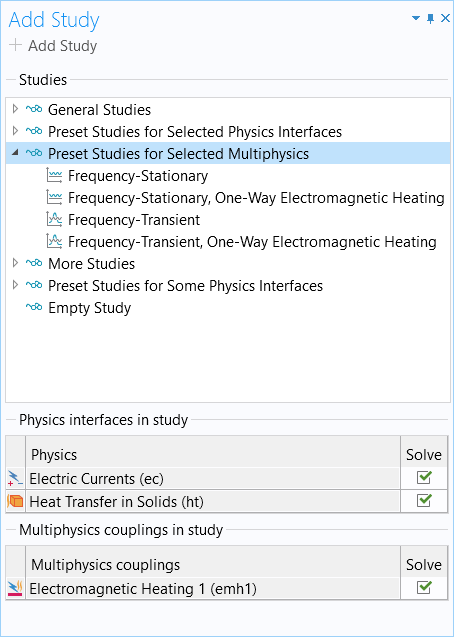# Learning Center Resources

## Setting Up and Solving Electromagnetic Heating Problems with High-Frequency Loads

There are some guidelines you can follow when working with a multiphysics problem involving electromagnetics and heat transfer, where:

• The electromagnetic fields are varying at a constant frequency, set of distinct frequencies, or a frequency that is very slowly varying compared to the period
• The period is very short compared to the timespan over which the system heats up
• The electrical properties are a function of the changing temperature over time

Let's address how you should set up and compute such models efficiently.

### Background

Many multiphysics problems involve physics that have different characteristic time scales. One of the most common cases is electrothermal heating. For example, consider a heater connected to the electrical grid. The applied voltage is varying at 60 Hz, but you may want to simulate the heating over several minutes or longer. In addition, the material properties are temperature dependent, thus the electromagnetic field solution will vary as the system heats up. However, solving for both of these governing equations in the time domain would be very time consuming, and is almost certainly unnecessary.

### Model Setup and Computation

When the electromagnetic loads and solution fields are sinusoidally varying (or very nearly so) over a single cycle, then you do not need to solve a time-domain problem for that physics. Instead, the electromagnetic fields can be solved in the frequency domain, which assumes that the material properties are constant over one cycle and that the solution fields are time harmonic. Under these assumptions, the electromagnetic physics can be solved as a stationary problem with relatively little computational effort, while still solving for the time evolution of the temperature fields.

To learn more about how COMSOL Multiphysics® handles problems in the frequency domain, please see the respective Learning Center article. There is also a blog post that outlines guidance on which study types to use for an electrothermal analysis.The Multiphysics branch with the Electromagnetic Heating multiphysics coupling feature, which considers the cycle-averaged loads.

To conduct the analysis, first, you must have an Electromagnetic Heating multiphysics coupling under the Multiphysics branch of your model tree, as shown in the screenshot above. Then, add a Frequency-Transient study type to your model. This study type is only available when the appropriate multiphysics coupling feature has already been added to the model.The various multiphysics study types that will solve a frequency-domain electromagnetics problem with a stationary or transient thermal problem.

If you instead want to make the simplifying assumption that the electromagnetic field solution does not depend upon the temperature field at all, then instead use the sequential frequency-transient study type, Frequency-Transient, One-Way Electromagnetic Heating. The study will first solve the electromagnetic problem, and then the transient heat transfer problem in a following study step.

If you are solving for the steady-state temperature fields, you can use the Frequency-Stationary study type. This study will incorporate the temperature-dependent material properties in the electromagnetic analysis. You can also use the sequential frequency-stationary study type, Frequency-Stationary, One-Way Electromagnetic Heating, to first solve the frequency-domain electromagnetic problem, and then the stationary thermal problem.